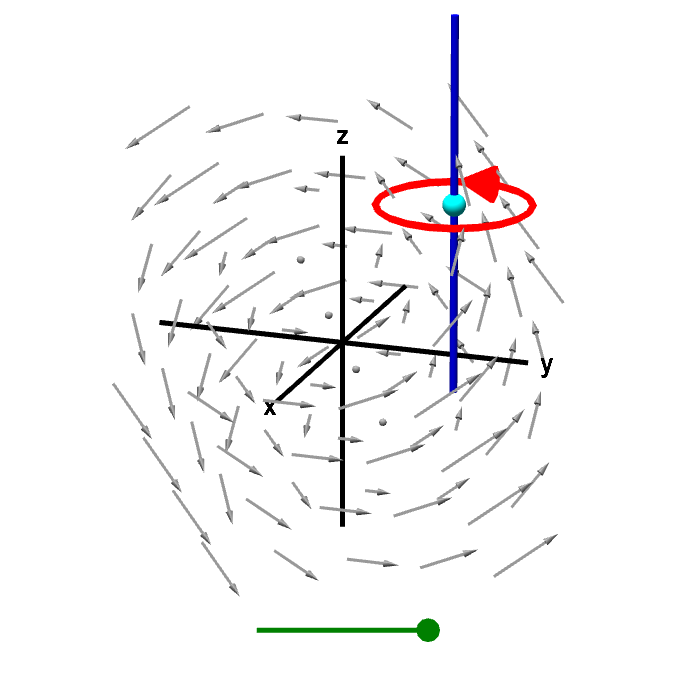# Math Insight

### Applet: A line integral gives z-component of curlA line integral around a curve in a plane perpendicular to the $z$-axis gives circulation of the vector field corresponding to the $z$-component of the curl. Dividing this line integral the the area of the region inside the curve, and letting the curve shrink to zero gives circulation per unit area. This circulation per unit area is the $z$-component of the curl. You can drag the green point on the slider to shrink the curve to a point or drag the cyan point to change the location where the $z$-component of the curl is being calculated.